# EC4217｜Linear LED Driver

Linear LED Driver

(Pre-limitary Version)

EC4217

GENERAL DESCRIPTION

EC4217 is a signal segment Linear LED Driver chip, Supply current(Sink) is 120mA at VLED=25V and Pow=15V. Other characteristic are sample application circuit (EC4217 use in 3.5 watts LED driver is only bridge rectifier and one 150kΩ resistor and one 4.7uF capacitor) and ​​ OVP( Over Voltage Protection)Function and negative coefficient ​​ output current behavior

FEATURES

TO-252-5L Package Type

Sample application circuit

Built in 8V~15V Zener Diode equal circuit to supply chip power which no need Start up HVNMOS, so is convenient used in 110V/220V line voltage

APPLICATIONS

LED Bulbs

ABSOLUTE MAXIMUM RATINGS

IZ=8mA

Pow=16.5V

Pin1 Break Down Voltage=600V at Pow=0V

Operating Temperature Range-40 to 120

Storage Temperature Range-40 to 140

ESD Level………………(H.B.M) 2KV

​​ ​​ ​​ ​​ ​​ ​​ ​​ ​​ ​​ ​​ ​​ ​​ ​​ ​​ ​​ ​​ ​​ ​​ ​​ ​​ ​​ ​​ ​​ ​​ ​​ ​​ ​​ ​​ ​​ ​​ ​​ ​​ ​​ ​​ ​​ ​​ ​​ ​​ ​​ ​​ ​​​​ (M.M) 200V

Note:

Absolute Maximum Ratings are those values beyond which damage to the device may occur. Functional Operation under these conditions is not implied. Continuous operation of the device at the absolute rating level

may affect device reliability

PIN CONFIGURATION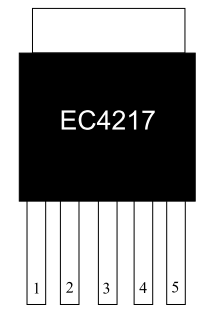Top View

 Pin Number Pin Name Function 1 LED Link LED 2 OVP_In Over Voltage Detect In 3 Gnd Ground 4 Vref 2.5V Reference Voltage 5 Pow Chip Power Supply

Ordering Information

 Part No.  ​​ ​​​​ Package Type  ​​​​ Marking Information Remark EC4217NNA5R TO-252-5L EC4217LLLLLYYWWT LLLLL：Lot No YYWW：Date CodeT：Internal Tracking Code

ELECTRICAL CHARACTERISTICS

TA = 25°C unless otherwise specified

 Parameter Symbol Condition Min Typ Max Unit Power Pow R1=30K, VCC=30V, Pin4 Open 8.25 8.36 8.51 V Reference Voltage Vref Pin4 Open 2.54 2.59 2.64 V Leak Current ILeak Pow=0V, V(LED)=40V 1.25 uA Driver Current ID Pow=15V, VLED=25V 110 mA Quiescent Current IQ R1=150K, Pin1 Open, Vi=110Vrms 0.75 mArms Over Voltage Protection OVP R3=820K, Pin1 Voltage 82 92 108 V Vref Input Resistor Ri Pow Open, Pin2 to Gnd Resistor 88 kΩ Channel Resistor RON VCC=30V, R1=22K, C1=4.7uF 94 Ω

Functional Description

EC4217 is sample application circuit 、low cost 、high stability signal segment Linear LED Driver. ​​ The EC4217 power setup please reference (Fig1), The application circuit external resistor ‘R2’ use to ​​ setup chip operation voltage and drive current , The operation voltage and drive current is calculated by formula 1. ​​ Other define, The chip between Pin5(Pow) with Pin4(Vref) is resistor R9 , ​​ between Pin4(Vref) with Gnd is resistor R9A, The value are 192k and 88k (reference Fig2), ​​ The Pin4 has reference voltage 2.58V.

Pow=2.58V+2.58VR9R size 12{"Pow"=2 "." 58V+2 "." 58V { {"R9"} over {R} } } {}

​​ ​​ ​​ ​​ ​​ ​​ ​​ ​​ ​​ ​​ ​​ ​​ ​​ ​​ ​​ ​​ ​​ ​​ ​​ ​​ ​​ ​​ ​​ ​​ ​​ ​​ ​​ ​​ ​​ ​​ ​​ ​​ ​​ ​​ ​​ ​​ ​​ ​​ ​​ ​​ ​​​​ ……Formula ​​ 1  ​​​​ R=R2(Chip external resistor)//R9A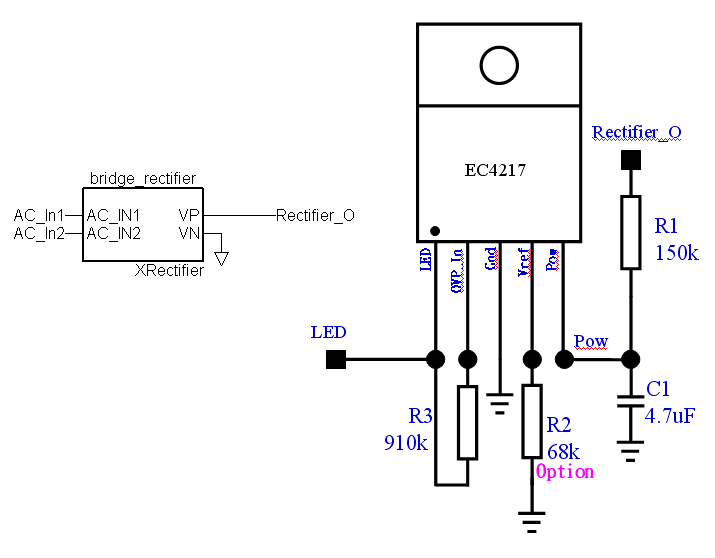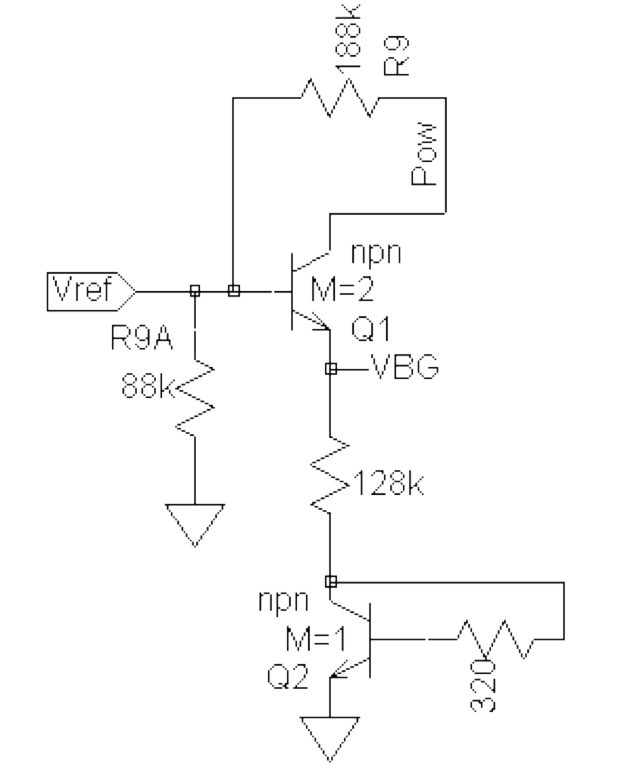​​ ​​ ​​ ​​ ​​ ​​ ​​ ​​ ​​ ​​ ​​ ​​ ​​ ​​ ​​ ​​ ​​ ​​ ​​ ​​ ​​ ​​ ​​ ​​ ​​ ​​ ​​ ​​ ​​ ​​ ​​ ​​ ​​ ​​ ​​ ​​ ​​ ​​ ​​ ​​ ​​ ​​ ​​ ​​ ​​ ​​ ​​ ​​ ​​ ​​​​ Fig1  ​​ ​​ ​​ ​​ ​​ ​​ ​​ ​​ ​​ ​​ ​​ ​​ ​​ ​​ ​​ ​​ ​​ ​​ ​​ ​​ ​​ ​​ ​​ ​​ ​​ ​​ ​​ ​​ ​​ ​​ ​​ ​​ ​​ ​​ ​​ ​​ ​​ ​​ ​​ ​​ ​​ ​​ ​​ ​​ ​​ ​​ ​​ ​​ ​​ ​​ ​​ ​​ ​​ ​​ ​​ ​​ ​​ ​​ ​​ ​​ ​​ ​​ ​​ ​​ ​​ ​​ ​​​​ Fig2

LED drive current(VLED=25V)setup reference Table 1,

 Pow(V) ID(I LED mA) ±2.5% 15 135 14.5 131 14 128.5 13.5 121 13 115 12.5 107 12 99.5 11.5 92 11 85.5 10.5 79.5 10 73 9.5 66 9 60 8.5 52.5 8 46.5

​​ ​​ ​​ ​​ ​​ ​​ ​​ ​​ ​​ ​​ ​​ ​​ ​​ ​​ ​​ ​​ ​​ ​​ ​​ ​​ ​​ ​​ ​​ ​​ ​​ ​​ ​​ ​​ ​​ ​​ ​​ ​​ ​​ ​​ ​​ ​​ ​​ ​​ ​​ ​​ ​​ ​​ ​​ ​​ ​​ ​​ ​​ ​​ ​​ ​​ ​​ ​​ ​​ ​​ ​​ ​​ ​​ ​​ ​​ ​​ ​​ ​​ ​​ ​​ ​​ ​​ ​​ ​​​​ Table 1

OVP Set up: Fig 3 is chip Pin4 (OVP_In) equal circuit, When OVP_In13V start OVP function , After OPV function is enabled then Pow(Pin5)0V, The OVP_IN(V) is calculated by formula 2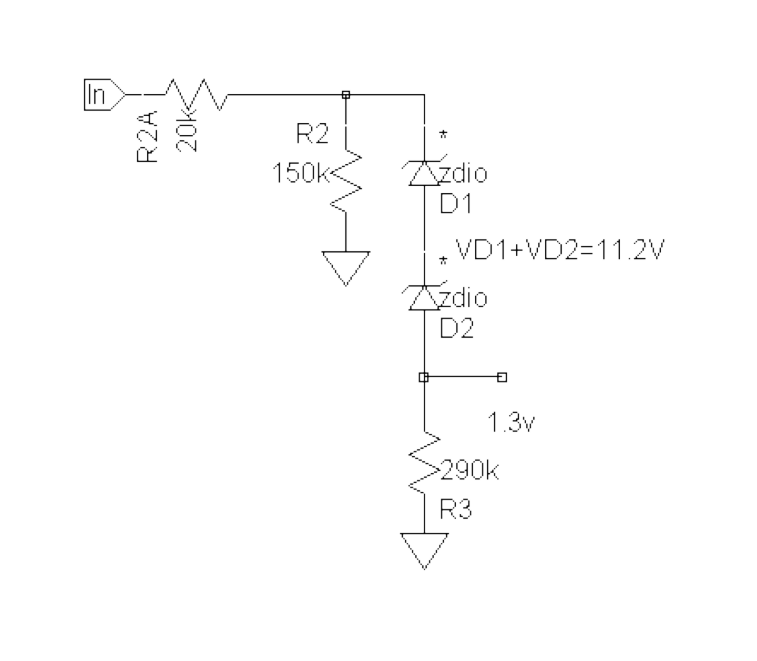OVP_IN(V)=R2A+R2R2A+R2+R3(Rext)Pin1(V)..........2 size 12{"OVP_IN" $$V$$ = { {"R2A"+"R2"} over {"R2A"+"R2"+"R3" $$"Rext"$$ } } cdot "Pin1" $$V$$ "." "." "." "." "." "." "." "." "." "." 2} {}

​​ ​​ ​​ ​​ ​​ ​​ ​​ ​​ ​​ ​​ ​​ ​​ ​​ ​​ ​​ ​​ ​​ ​​ ​​ ​​ ​​ ​​ ​​ ​​ ​​ ​​ ​​ ​​​​ Fig3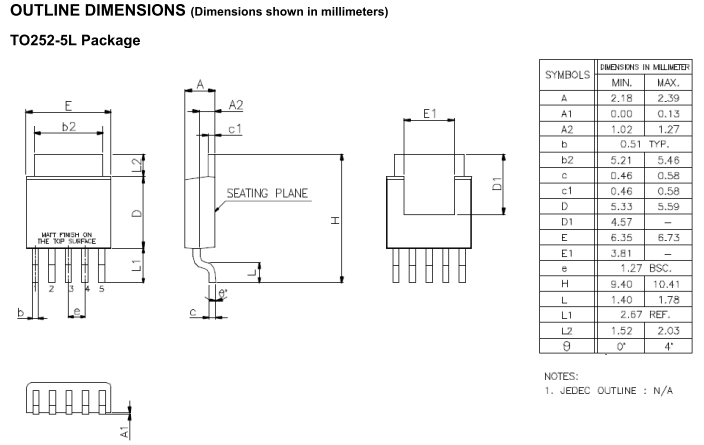### 关于飞虹高科